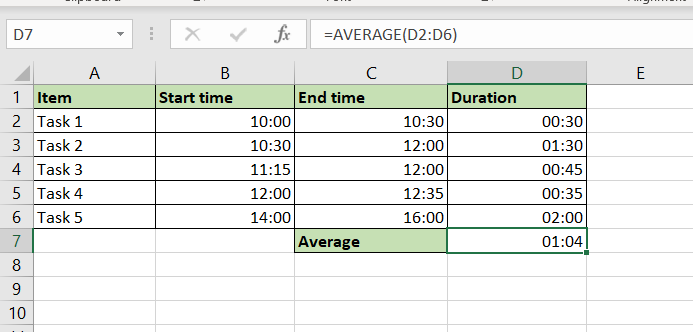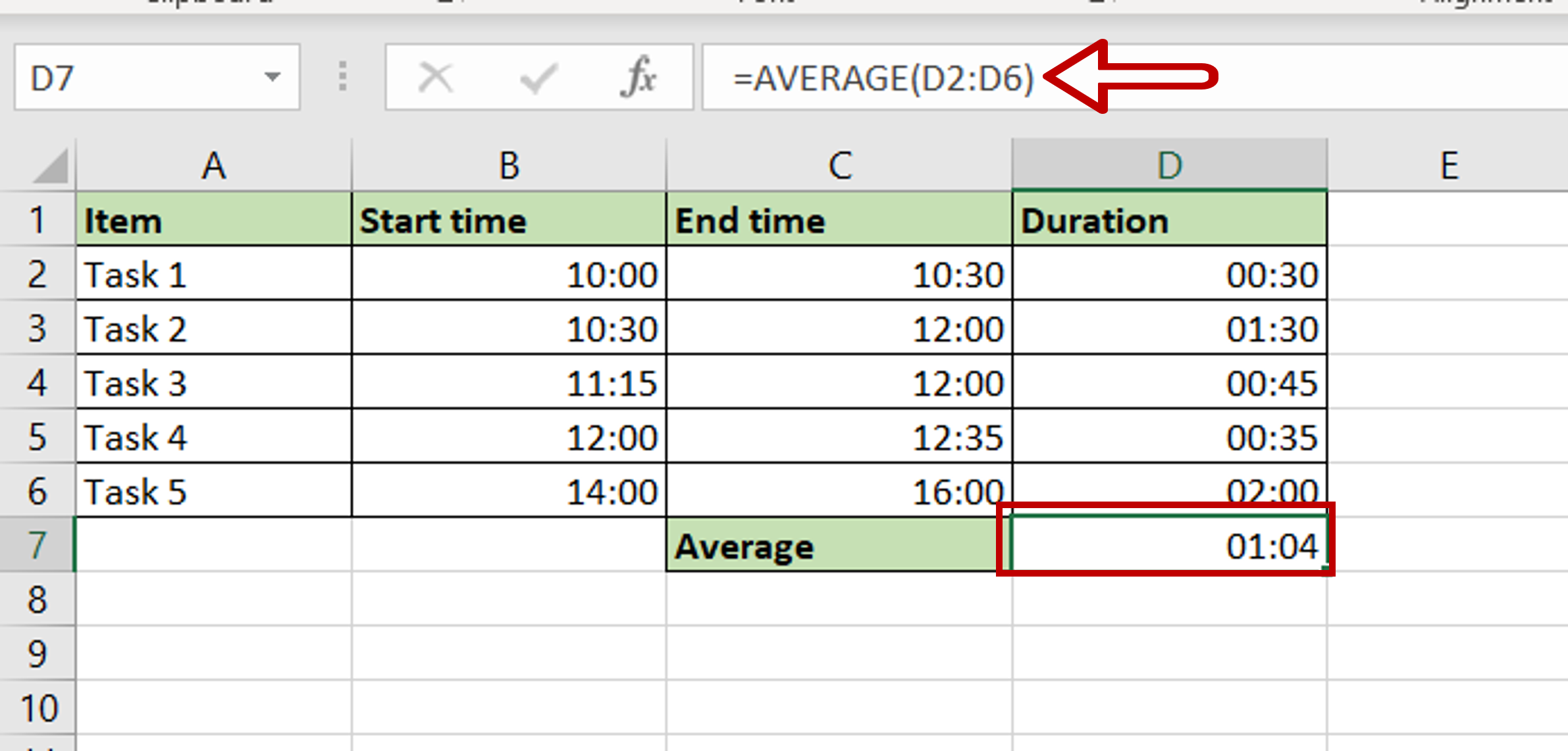# How to calculate average time in Excel

You can watch a video tutorial here.Excel treats date and time formats differently from numbers and text. Because of the different scales i.e. 24 hours, 60 minutes, and 60 seconds, when adding minutes and seconds they cannot be treated like regular numbers. In Excel, to be able to add or subtract dates and times, you need to format them as dates and times for the operation to work correctly. With this format, you will be able to use regular mathematical operators and functions.

### Step 1 – Create the formula– Select the cell where the result is to appear
– Type the formula using cell references:
=AVERAGE(Duration)

### Step 2 – Check the result– The average Duration is displayed as hours and minutes